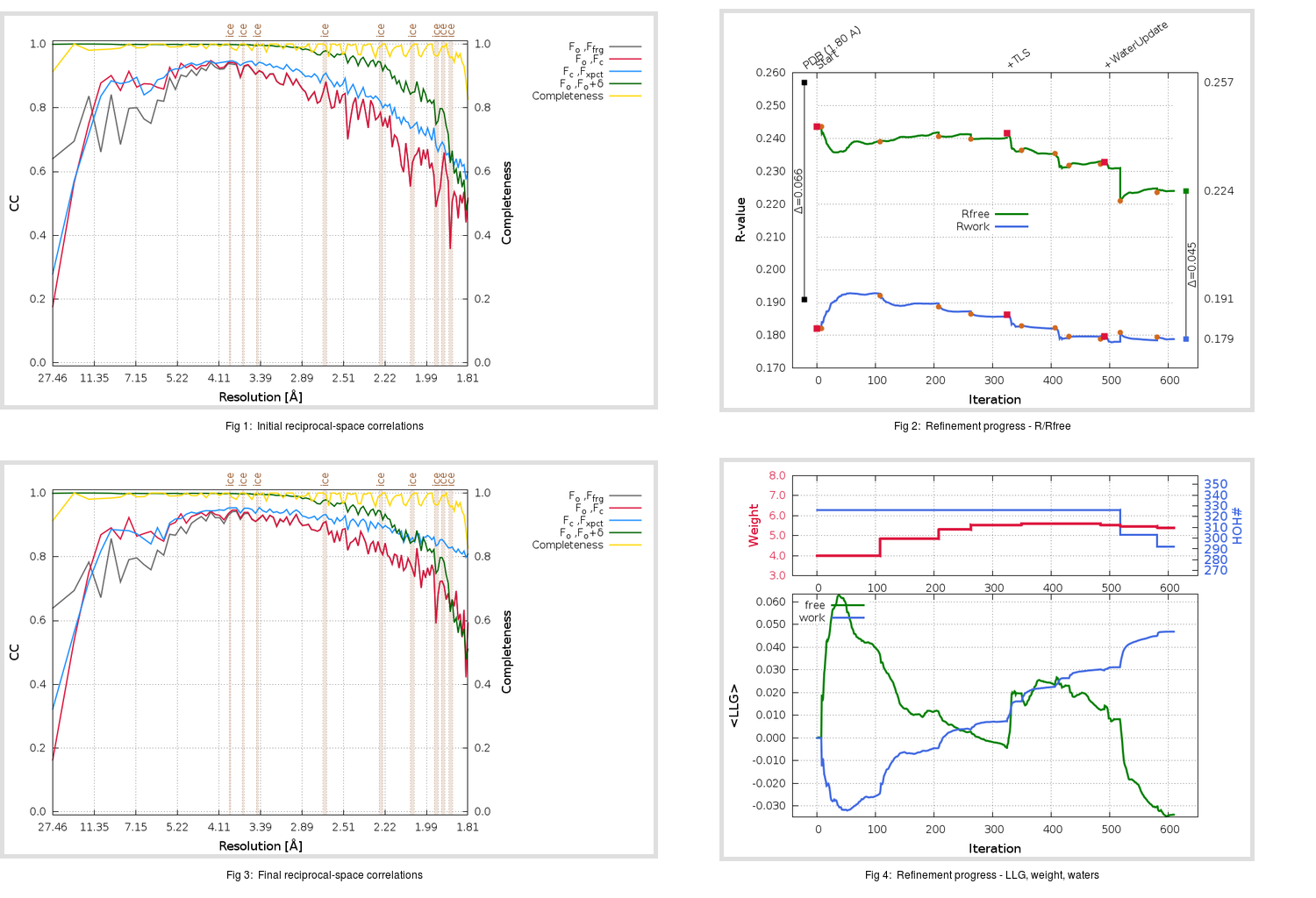Content:

```    Diffraction limits & principal axes of ellipsoid fitted to diffraction cut-off surface:
1.793         0.9556   0.0000   0.2946       a* + 0.029 c*
1.746         0.0000   1.0000   0.0000       b*
1.736        -0.2946   0.0000   0.9556      -0.606 a* + 0.795 c*
```

## Deposited

` `
 Date deposited Date data collection Resolution R, Rfree 20200315 20200227 1.80 0.1870 0.2570

Molprobity (CCP4 7.0 version) summary:

```Ramachandran outliers =   0.66 %
favored =  96.69 %
Rotamer outliers      =   1.89 %
C-beta deviations     =     0
Clashscore            =   8.43
RMS(bonds)            =   0.0140
RMS(angles)           =   1.90
MolProbity score      =   1.88
Resolution            =   1.80
R-work                =   0.1870
R-free                =   0.2570
```

```Number of waters      =   326

<B> (all atoms) =   29.15 ( sd =   10.06 ) for       2730 non-hydrogen atoms
<B>   (protein) =   27.79 ( sd =    9.23 ) for       2370 non-hydrogen atoms
<B>     (water) =   38.20 ( sd =   10.47 ) for        326 non-hydrogen atoms
<B>    (others) =   36.42 ( sd =   13.08 ) for         34 non-hydrogen atoms

B min/max       (all non-hydrogen atoms) =   15.80 /  103.23
B min/max   (protein non-hydrogen atoms) =   16.19 /   91.56
B min/max     (water non-hydrogen atoms) =   15.80 /  103.23
B min/max     (other non-hydrogen atoms) =   26.44 /   63.47
```

## BUSTER (re-)refinement

` `

Molprobity (CCP4 7.0 version) summary:

```Ramachandran outliers =   0.33 %
favored =  97.68 %
Rotamer outliers      =   0.76 %
C-beta deviations     =     0
Clashscore            =   3.16
RMS(bonds)            =   0.0119
RMS(angles)           =   1.60
MolProbity score      =   1.18
Resolution            =   1.80
R-work                =   0.1790
R-free                =   0.2241
```

```Number of waters      =   292

<B> (all atoms) =   30.15 ( sd =    8.57 ) for       2696 non-hydrogen atoms
<B>   (protein) =   28.63 ( sd =    7.08 ) for       2370 non-hydrogen atoms
<B>     (water) =   41.07 ( sd =    9.99 ) for        292 non-hydrogen atoms
<B>    (others) =   41.33 ( sd =   14.04 ) for         34 non-hydrogen atoms

B min/max       (all non-hydrogen atoms) =   16.79 /   73.66
B min/max   (protein non-hydrogen atoms) =   17.84 /   73.66
B min/max     (water non-hydrogen atoms) =   16.79 /   68.65
B min/max     (other non-hydrogen atoms) =   28.99 /   67.66
```

Refinement progression:Results:

` `
 File Remark 5REH_aB_refine.01_03_refine.pdb.gz exact refinement commands are in header 5REH_aB_refine.01_03_refine.mtz.gz including original deposited data and several re-refinement map coefficients 5REH_aB_refine.01_03_BUSTER_model.cif.gz including any non-standard compound restraints 5REH_aB_refine.01_03_BUSTER_refln.cif.gz